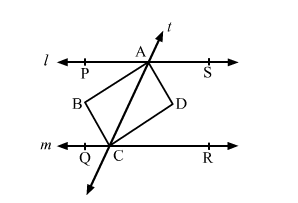# Two parallel lines l and m are intersected by a transversal t.

Question:

Two parallel lines l and m are intersected by a transversal t. Show that the quadrilateral formed by the bisectors of interior angles is a rectangle.

Solution:Given: l || m and the bisectors of interior angles intersect at and D.

To prove: ABCD is a rectangle.

Proof:

Since,
l || m                       (Given)

So, $\angle P A C=\angle A C R$  (Alternate interior angles)

$\Rightarrow \frac{1}{2} \angle P A C=\frac{1}{2} \angle A C R$

$\Rightarrow \angle B A C=\angle A C D$

but, these are a pair of alternate interior angles for AB and DC.

$\Rightarrow A B \| D C$

Similarly, $B C \| A D$

So, ABCD is a parallelogram.

Also,

$\angle P A C+\angle C A S=180^{\circ} \quad$ (Linear pair)

$\Rightarrow \frac{1}{2} \angle P A C+\frac{1}{2} \angle C A S=90^{\circ}$

$\Rightarrow \angle B A C+\angle C A D=90^{\circ}$

$\Rightarrow \angle B A D=90^{\circ}$

But, this an angle of the parallleogram ABCD.

Hence, ABCD is a rectangle.